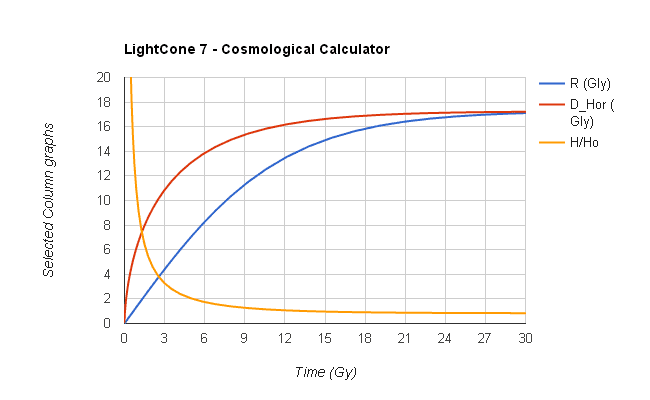# At what point would we stop seeing a star?

• B
The universe is expanding and at some point faster than light so once it reaches that point we wont see the light from those stars. I forget what this point is called.

If someone happened to be watching a star as it crossed that point would we see it simply blink out or close to that or would it kind of fade away to blackness. It seems to me that there should be a precise point where the speed of light is reached and one mile an hour faster than that would stop us from seeing it.

Of course since the star is huge the light from the leading edge of the star would be the first to go out then it would progress to the trailing edge so maybe it would not quite be a blink but close to it.

Am I thinking right?

tex

George Jones
Staff Emeritus
Gold Member
The universe is expanding and at some point faster than light so once it reaches that point we wont see the light from those stars. I forget what this point is called.

Care is needed with respect to the definition of "speed" in a cosmological setting.

this isn't true. It is true that recession speeds of galaxies that we now see will eventually exceed c, but it is not true that we loose sight of a galaxy once its recession speed exceeds c. If we see a galaxy now, then we will (in principle) always see the galaxy, even when its recession speed exceeds c.

Suppose we now see galaxy A. Assume that at time t in the future, A's recession speed is greater than c, and that at this time someone in galaxy A fires a laser pulse directly at us. Even though the pulse is fired directly at us, the proper distance between us and the pulse will initially increase. After a while, however, the pulse will "turn around", and the proper distance between us and the pulse will decrease, and the pulse will reach us, i.e., we still see galaxy A.

This can happen because the Hubble constant decreases with time (more on this near the end of this post) in the standard cosmological model for our universe. Consider the following diagram:

Code:
O                                B        A        C
*                                *        *        *

*                    *     *     *
O                    B     A     C

The bottom row of asterisks represents the positions in space (proper distances) of us (O) and galaxies B, A, and C, all at the same instant of cosmic time, $t_e$. The top row of asterisks represents the positions in space of us (O) and galaxies B, A, and C, all at some later instant of cosmic time, $t$. Notice that space has "expanded" between times $t_e$ and $t$.

Suppose that at time $t_e$: 1) galaxy A has recession speed (from us) greater than c; 2) galaxy A fires a laser pulse directed at us. Also suppose that at time $t$, galaxy B receives this laser pulse. In other words, the pulse was emitted from A in the bottom row and received by B in the top row. Because A's recession speed at time $t_e$ is greater than c, the pulse fired towards us has actually moved away from us between times $t_e$ and $t$.

Now, suppose that the distance from us to galaxy B at time $t$ is the same as the distance to galaxy C at time $t_e$. Even though the distances are the same, the recession speed of B at time $t$ is less than than the recession speed of C at time $t_e$ because:

1) recession speed equals the Hubble constant multiplied by distance;

2) the value of the Hubble constant decreases between times $t_e$ and $t$.

Since A's recession speed at time $t_e$ is greater than c, and galaxy C is farther than A, galaxy C's recession speed at time $t_e$ also is greater than c. If, however, the Hubble constant decreases enough between times $t_e$ and $t$, then B's recession speed at time $t$ can be less than c. If this is the case, then at time $t$ (and spatial position B), the pulse is moving towards us, i.e., the pulse "turned around" at some time between times $t_e$ and $t$.

If the value of the Hubble constant changes with time, what does the "constant" part of "Hubble constant" mean? It means constant in space. At time $t_e$, galaxies O, B, A, and C all perceive the same value for the Hubble constant. At time $t$, galaxies O, B, A, and C all perceive the same value for the Hubble constant. But these two values are different.

Probably some of my explanation is unclear. If so, please ask more questions.

Since the speed of recession is approaching the speed of light the star would be red-shifting. The horizon of the Universe behave very much like a black hole event horizon, the star would appear frozen at the horizon and red shift out of existence.

Jorrie
Gold Member
Since the speed of recession is approaching the speed of light the star would be red-shifting. The horizon of the Universe behave very much like a black hole event horizon, the star would appear frozen at the horizon and red shift out of existence.
This is not quite correct. The distance to the cosmological horizon D_Hor is larger than the Hubble radius R, where the recession rate equals c.They only approach each other in the far future, when H becomes constant. Distant stars were always moving through the Hubble radius and we will see them farther and farther away, just red-shifting out of detectability, but never "frozen" at the horizon.

#### Attachments

•|Glitch|
Jorrie
•